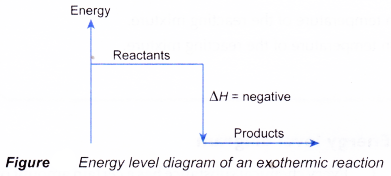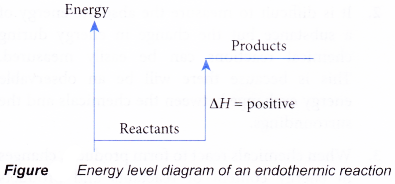# How does the energy level diagram show this reaction is exothermic?

## Energy profile diagrams for endothermic and exothermic reactions

• Every chemical substance has a certain amount of chemical energy. This energy is given the symbol H and is different for different substances.
• It is difficult to measure the absolute energy of a substance but the change in energy during chemical reactions can be easily measured. This is because there will be an observable energy exchange between the chemicals and the surroundings.
• When chemicals react to form products, changes in energy content of reactants and products occur. This will cause heat to be released or absorbed in the reaction.
• The amount of heat energy released or absorbed during a chemical reaction is called the heat of reaction.
• Heat of reaction is given the symbol AH and is usually measured in kilojoules (kJ).
ΔH = Total energy content of products – total energy content of reactants
= Hproducts – Hreactants
• In an exothermic reaction, the reactants lose heat energy to form products. Thus, the products formed have less energy than the reactants, Hproducts < Hreactants. Therefore, ΔH is negative.
• In an endothermic reaction, the reactants absorb heat energy from the surroundings to form products. Thus, the products formed have more energy than the reactans, Hproducts > Hreactants. Therefore, ΔH is positive.
• Energy level diagrams are used to shows the energy content of chemicals before and after a reaction. They show:
(a) the total energy content of the reactants compared to the total energy content of the products.
(b) the energy difference between the reactants and the products, ΔH, heat of reaction
• Figure shows the energy level diagram of an exothermic reaction.• Figure shows the energy level diagram of an endothermic reaction.• The following are the steps to construct energy level diagrams for exothermic and endothermic reactions.
 Steps What to do? 1 Identify whether the reaction is exothermic or endothermic 2 Draw and label the energy axis 3 Draw the energy level for the reactants and products 4 Draw an arrow from the reactants level to the products level 5 Write in the reactants and products based on the balanced equation 6 Label ΔH as positive or negative
• Figure shows the energy level diagram for the reaction between methane and oxygen.Based on Figure, the following information can be obtained.
(a) The reaction between methane and oxygen to form carbon dioxide and water is an exothermic reaction.
(b) During the reaction, the temperature of the mixture increases.
(c) The total energy of 1 mole of methane and 2 moles of oxygen is more than the total energy of 1 mole of carbon dioxide and 2 moles of water by 890 kJ.
(d) Therefore, when 1 mole of methane reacts with 2 moles of oxygen to form 1 mole of carbon dioxide and 2 moles of water, 890 kJ of heat is produced.
CH4(g) + 2O2(g) → CO2(g) + 2H2O(l)     ΔH = -890 kJ
• Figure shows the energy level diagram for the thermal decomposition of calcium carbonate.Based on Figure, the following information can be obtained.
(a) The thermal decomposition of calcium carbonate to calcium oxide and carbon dioxide is an endothermic reaction.
(b) During the decomposition, the temperature of the mixture decreases.
(c) The total energy of 1 mole of calcium carbonate is less than the total energy of 1 mole of calcium oxide and 1 mole of carbon dioxide by 570 kJ.
(d) Therefore, when 1 mole of calcium carbonate decomposes to form 1 mole of calcium oxide and 1 mole of carbon dioxide, 570 kJ of heat is absorbed.
CaCO3(s) → CaO(s) + CO2(g)    ΔH = +570 kJ